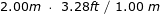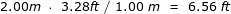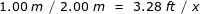# Applying Dimensional Analysis to Derive Units, Formulas & Solutions

Instructor: Michael Eckert

Michael has a Bachelor's in Environmental Chemistry and Integrative Science. He has extensive experience in working with college academic support services as an instructor of mathematics, physics, chemistry and biology.

Dimensional Analysis is a mathematical operation or series of operations used in sciences such as chemistry and physics. It is essentially the process of keeping track of and converting between units of measurement. Coupled with a little algebraic manipulation we can change from one unit of measurement to the next with relative ease.

## Applications of Dimensional Analysis

We may have some home improvement project, which requires that we find a distance in meters (when all we have is a yardstick. Similarly, we may be pressured into picking up an exact gallon of a food product at the grocery store (to find that the product is only sold in liters). This is where the process of dimensional analysis can be helpful.

## What is Dimensional Analysis?

Whether a unit of measurement is in the metric system (e.g. centimeters or meters) or the English system of weights and measures (e.g. inches or feet), it can be converted interchangeably between the two systems within its respective dimension of measurement. This applies to measurements of length, mass, temperature, speed and a host of other measurable values. Historically, the process of dimensional analysis has also been referred to as the Factor-Label Method or Unit-Factor Method. By keeping track of and by labeling units of measurement and by realizing that any number, variable or expression can be multiplied by 1 without changing its value, we can convert from one unit of measurement to the next easily and without error.

#### A One-Step Example of Dimensional Analysis

For the purposes of this lesson, let's use a straight-forward one-step example of dimensional analysis. If we wish to convert say 2.00 meters (m) a metric unit to feet (ft) an English unit, we must first establish that 1.00 meter (m) = 3.28 feet (ft.). To convert 2.00 m to ft, we set up the following:There are two things I want to point out in this equation:

1. Notice how we merely multiplied 2.00 m by 1 (in the form of 3.28 ft / 1.00 m).
2. Notice how we set up the proportion so that when simplified, m / m is reduced to 1, and we are simply left with 2.00 (3.28 ft) = 6.56 ft. To recap, without changing the actual quantity of distance, we changed its unit of measure from meters to feet by A) multiplying 2.00 m by 1 (i.e. 3.28 ft / 1.00 m) and by B) simplifying the expression.We can check that the dimensional analysis performed above indeed works by setting up a simple proportion and deriving an easy-to-solve algebraic formula for finding the number of feet in 2.00 meters. Given a known number of 2.00 m, let's find the corresponding length in feet. Again, we know that every 1.00 m = 3.28 ft, so we can set up the following proportion:where x will be the number of feet we are looking for. Simplifying, we get x (1/2) = 3.28 ft. Notice that m / m in our expression is reduced to 1 as it was above. Solving for x, we find that x = 6.56 ft, the same as above. Therefore, the dimensional analysis employed above checks out. Now we know how to do a one-step unit conversion using dimensional analysis.

To unlock this lesson you must be a Study.com Member.

### Register to view this lesson

Are you a student or a teacher?